# RD Sharma Solutions For Class 7 Maths Exercise 8.3Chapter 8 Linear Equations in One Variable

RD Sharma Solutions for Class 7 Maths Exercise 8.3 of Chapter 8 Linear Equation in One Variable are provided in simple PDF format. Learners can easily download this PDF from given links. BYJU’S experts have solved the questions present in this exercise, and this will help them with their exam point of view. Here we are suggested to practice RD Sharma Solutions for Class 7 to gain Math skills. This exercise discusses with transposition method, defined as any term of the equation can be taken to the other side with its sign changed without affecting the equality.

## Download the PDF of RD Sharma Solutions For Class 7 Maths Chapter 8 – Linear Equations in One variable Exercise 8.3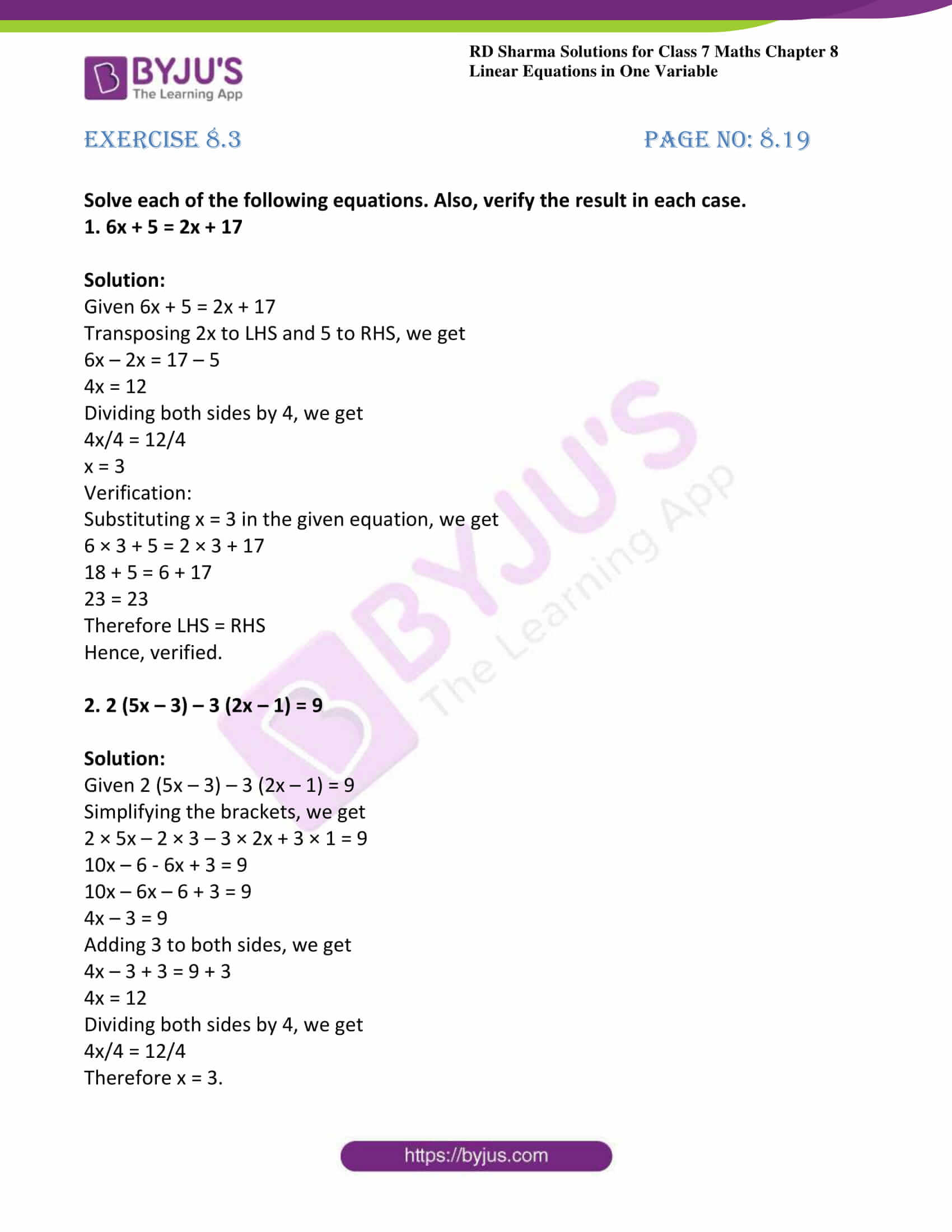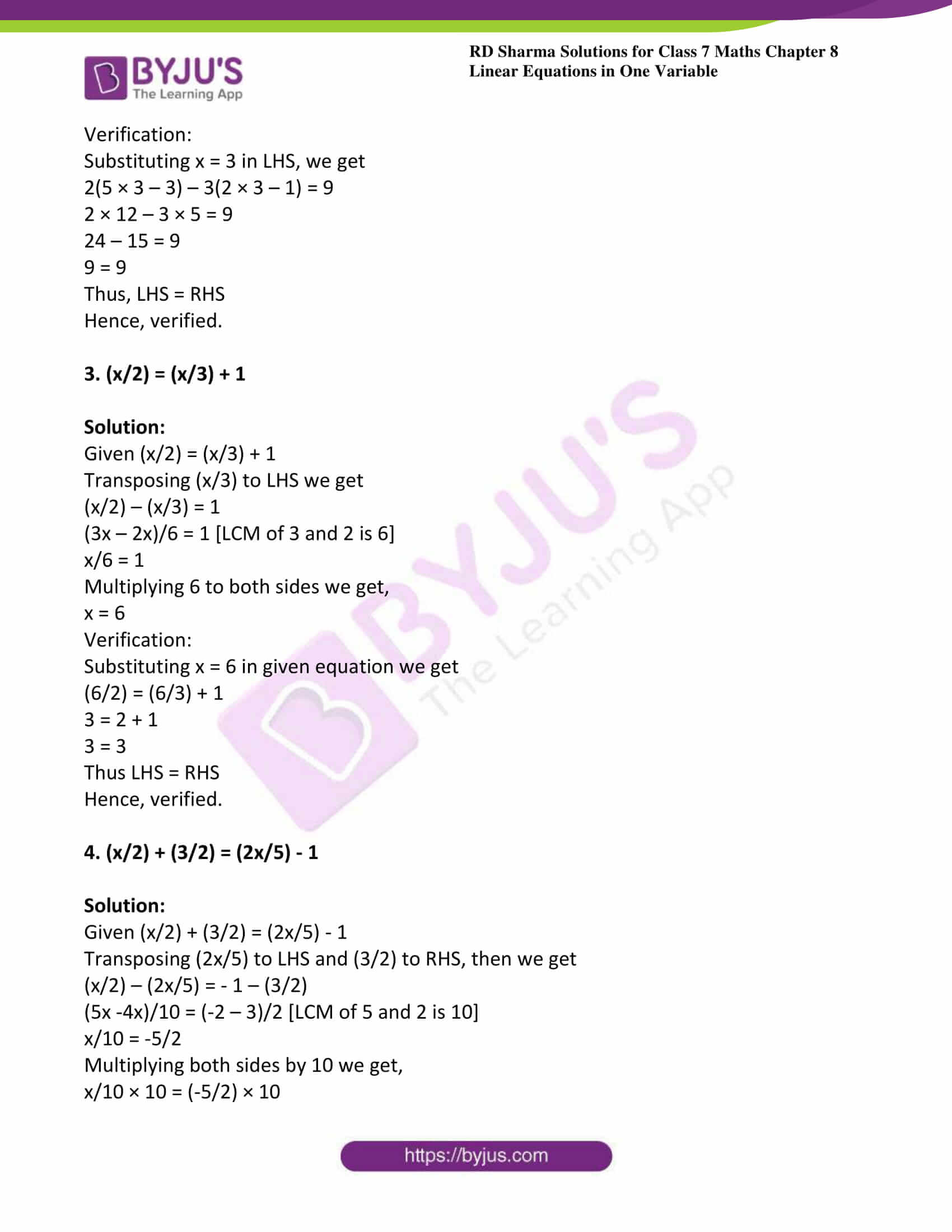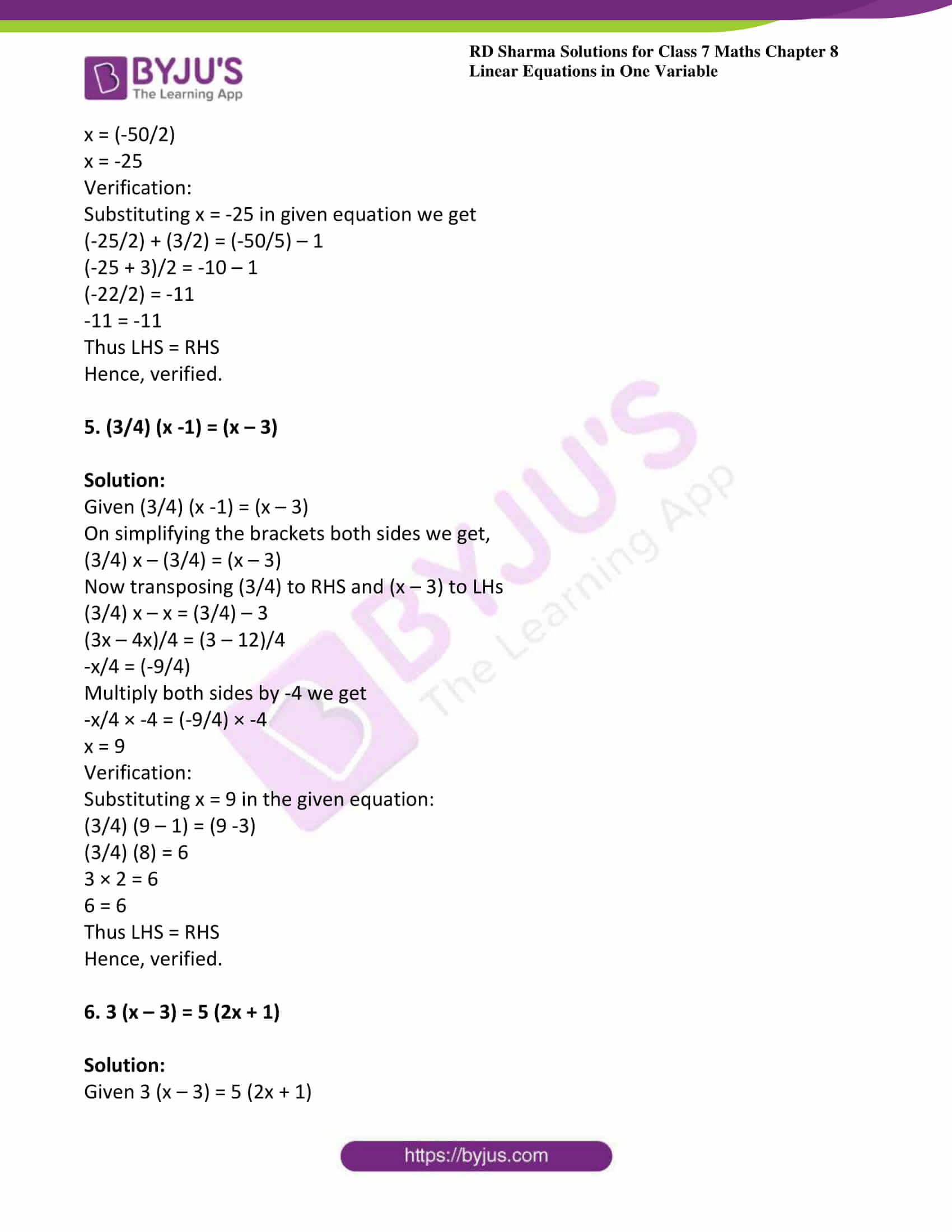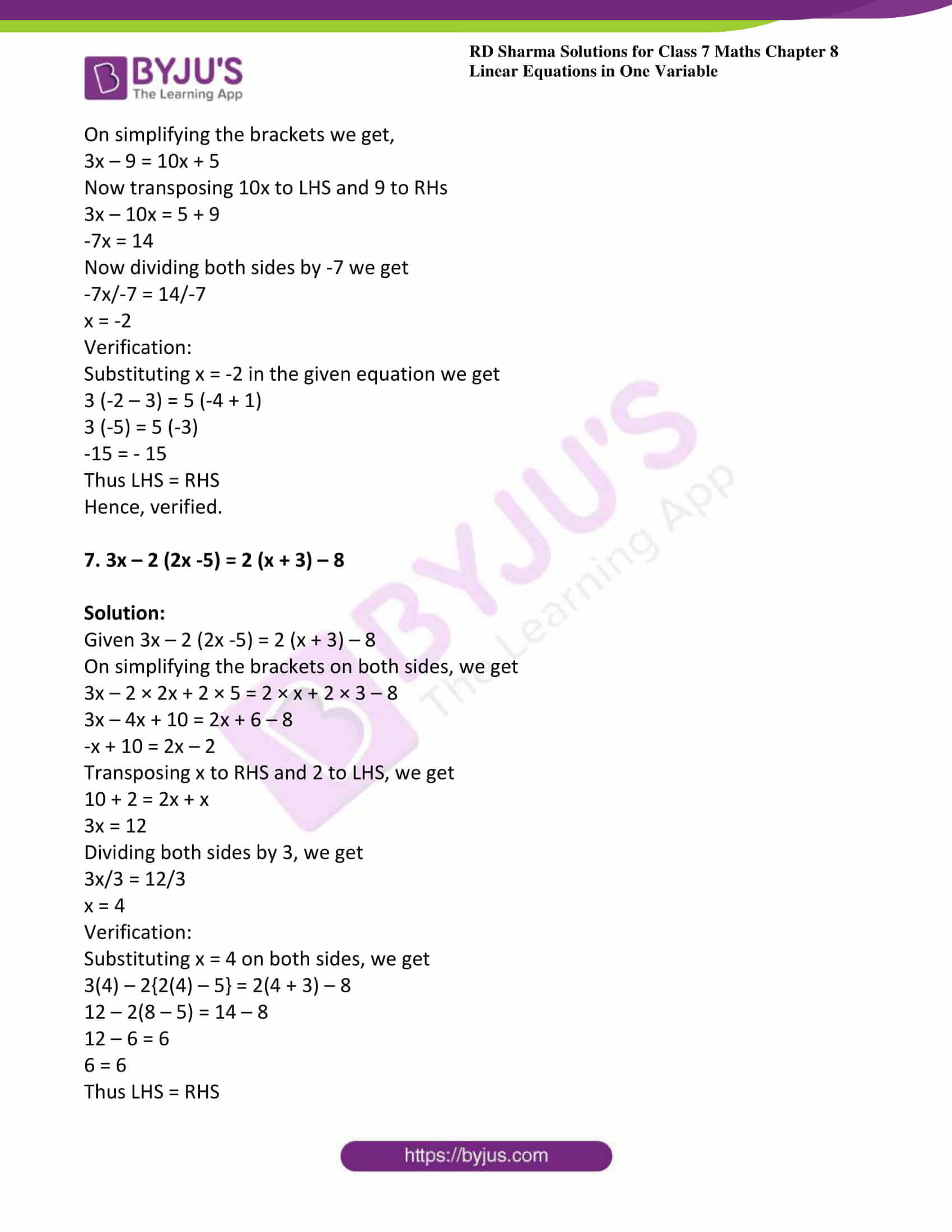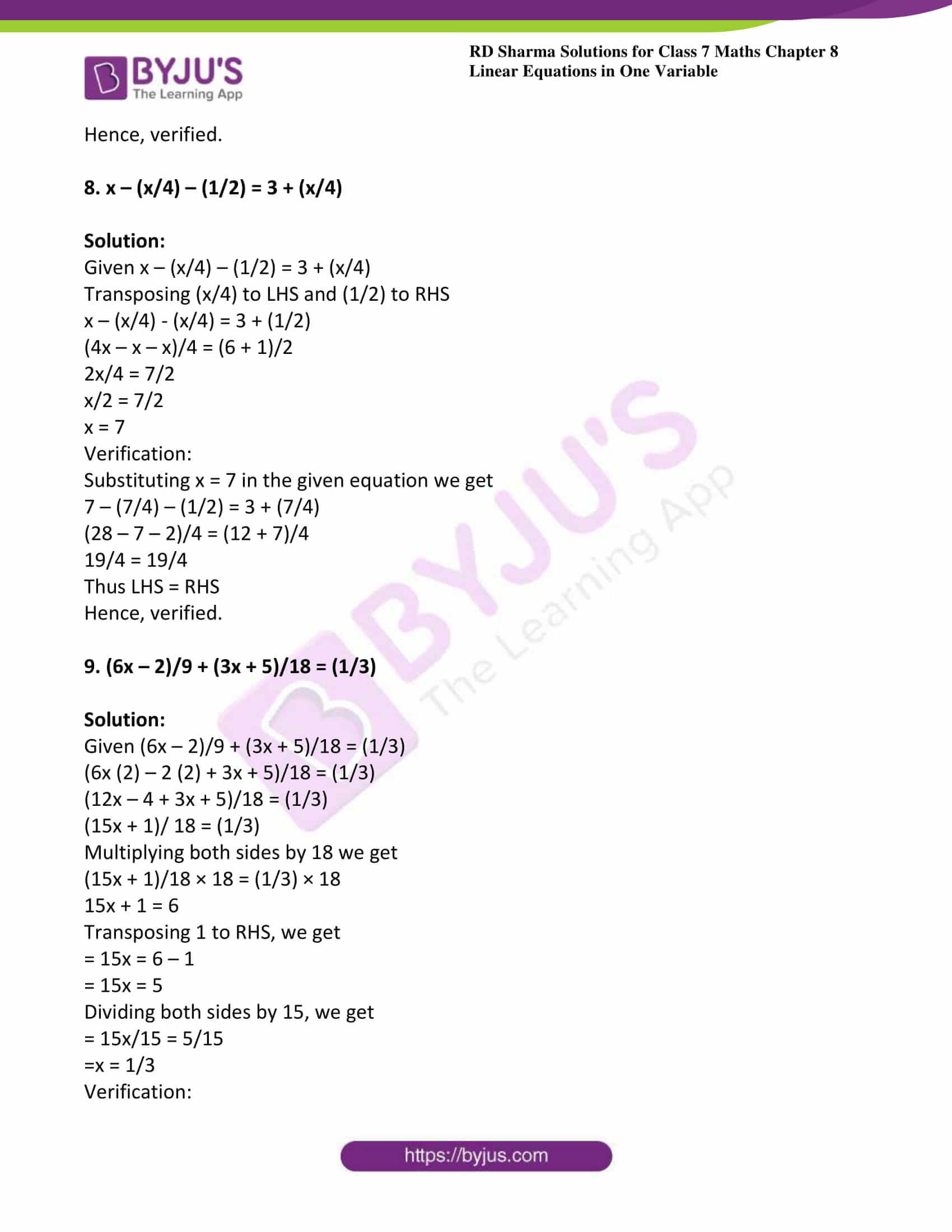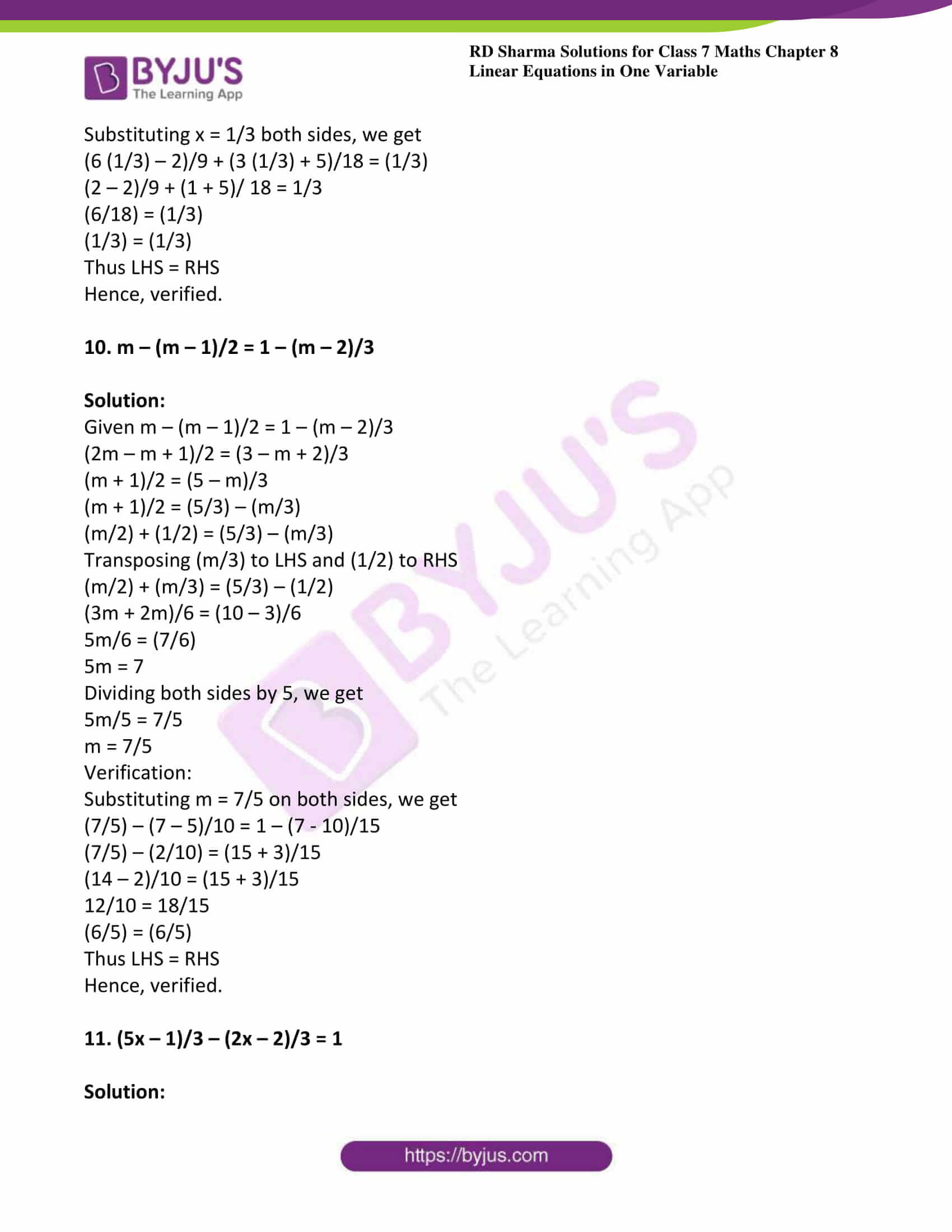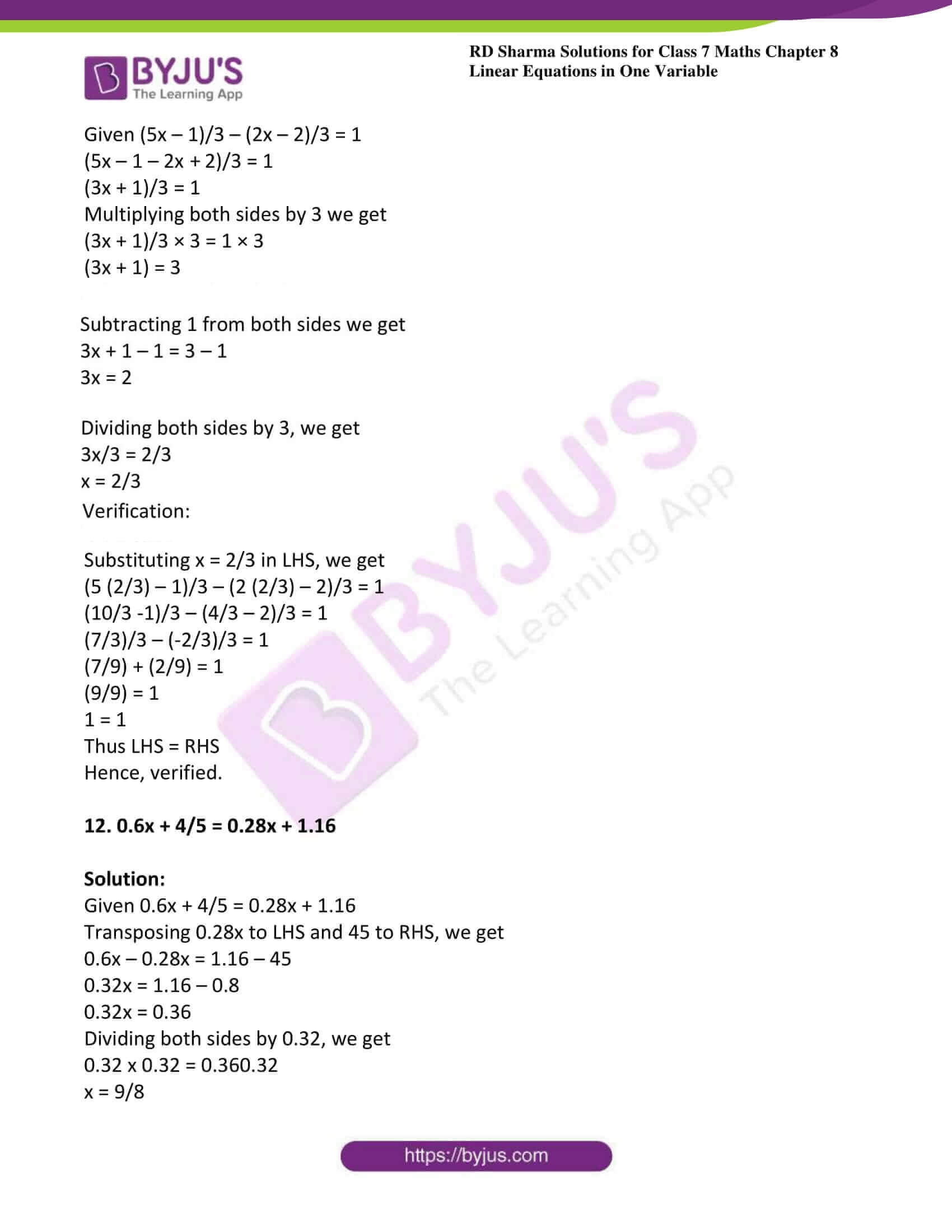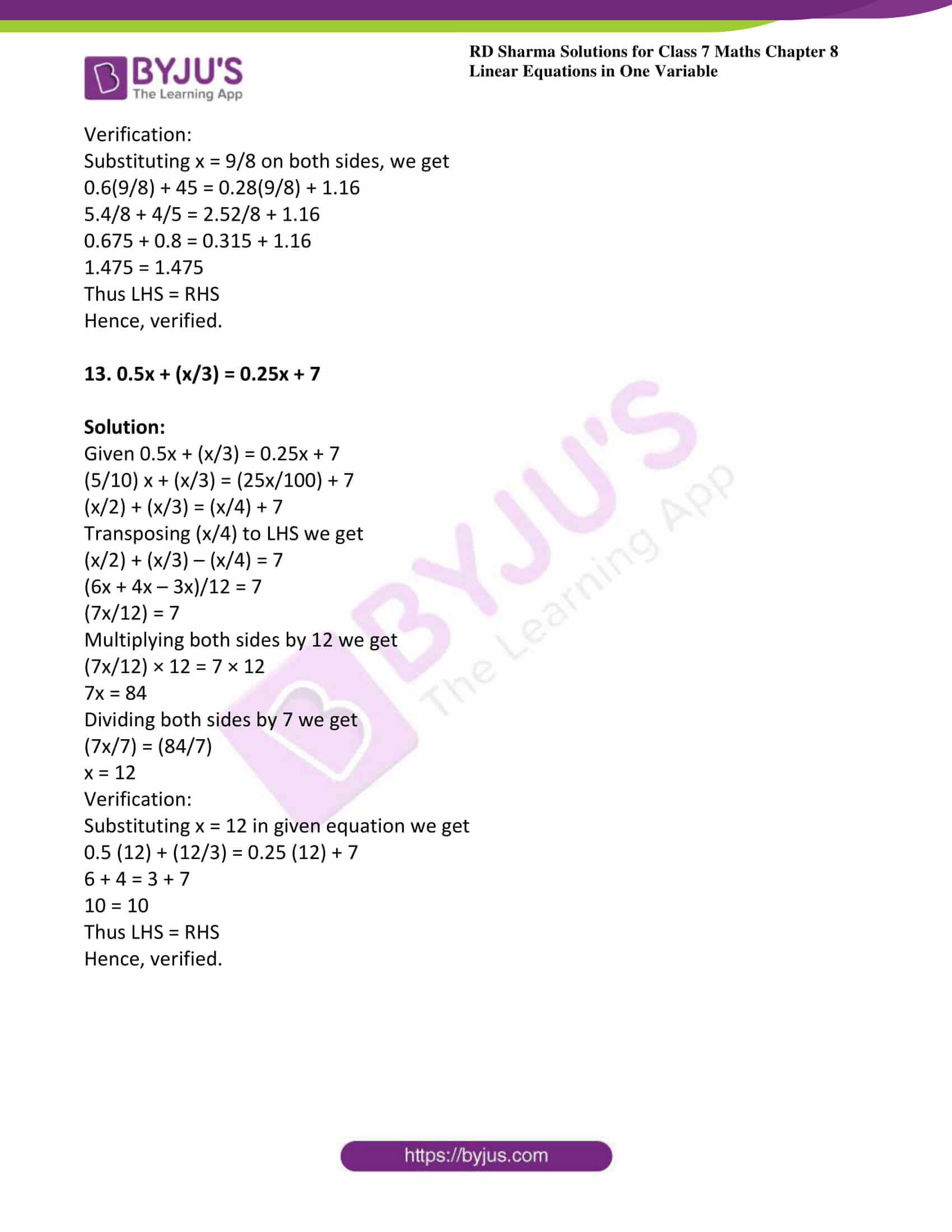### Access answers to Maths RD Sharma Solutions For Class 7 Chapter 8 – Linear Equation in One Variable Exercise 8.3

Solve each of the following equations. Also, verify the result in each case.

1. 6x + 5 = 2x + 17

Solution:

Given 6x + 5 = 2x + 17

Transposing 2x to LHS and 5 to RHS, we get

6x – 2x = 17 – 5

4x = 12

Dividing both sides by 4, we get

4x/4 = 12/4

x = 3

Verification:

Substituting x = 3 in the given equation, we get

6 × 3 + 5 = 2 × 3 + 17

18 + 5 = 6 + 17

23 = 23

Therefore LHS = RHS

Hence, verified.

2. 2 (5x – 3) – 3 (2x – 1) = 9

Solution:

Given 2 (5x – 3) – 3 (2x – 1) = 9

Simplifying the brackets, we get

2 × 5x – 2 × 3 – 3 × 2x + 3 × 1 = 9

10x – 6 – 6x + 3 = 9

10x – 6x – 6 + 3 = 9

4x – 3 = 9

Adding 3 to both sides, we get

4x – 3 + 3 = 9 + 3

4x = 12

Dividing both sides by 4, we get

4x/4 = 12/4

Therefore x = 3.

Verification:

Substituting x = 3 in LHS, we get

2(5 × 3 – 3) – 3(2 × 3 – 1) = 9

2 × 12 – 3 × 5 = 9

24 – 15 = 9

9 = 9

Thus, LHS = RHS

Hence, verified.

3. (x/2) = (x/3) + 1

Solution:

Given (x/2) = (x/3) + 1

Transposing (x/3) to LHS we get

(x/2) – (x/3) = 1

(3x – 2x)/6 = 1 [LCM of 3 and 2 is 6]

x/6 = 1

Multiplying 6 to both sides we get,

x = 6

Verification:

Substituting x = 6 in given equation we get

(6/2) = (6/3) + 1

3 = 2 + 1

3 = 3

Thus LHS = RHS

Hence, verified.

4. (x/2) + (3/2) = (2x/5) – 1

Solution:

Given (x/2) + (3/2) = (2x/5) – 1

Transposing (2x/5) to LHS and (3/2) to RHS, then we get

(x/2) – (2x/5) = – 1 – (3/2)

(5x -4x)/10 = (-2 – 3)/2 [LCM of 5 and 2 is 10]

x/10 = -5/2

Multiplying both sides by 10 we get,

x/10 × 10 = (-5/2) × 10

x = (-50/2)

x = -25

Verification:

Substituting x = -25 in given equation we get

(-25/2) + (3/2) = (-50/5) – 1

(-25 + 3)/2 = -10 – 1

(-22/2) = -11

-11 = -11

Thus LHS = RHS

Hence, verified.

5. (3/4) (x -1) = (x – 3)

Solution:

Given (3/4) (x -1) = (x – 3)

On simplifying the brackets both sides we get,

(3/4) x – (3/4) = (x – 3)

Now transposing (3/4) to RHS and (x – 3) to LHs

(3/4) x – x = (3/4) – 3

(3x – 4x)/4 = (3 – 12)/4

-x/4 = (-9/4)

Multiply both sides by -4 we get

-x/4 × -4 = (-9/4) × -4

x = 9

Verification:

Substituting x = 9 in the given equation:

(3/4) (9 – 1) = (9 -3)

(3/4) (8) = 6

3 × 2 = 6

6 = 6

Thus LHS = RHS

Hence, verified.

6. 3 (x – 3) = 5 (2x + 1)

Solution:

Given 3 (x – 3) = 5 (2x + 1)

On simplifying the brackets we get,

3x – 9 = 10x + 5

Now transposing 10x to LHS and 9 to RHs

3x – 10x = 5 + 9

-7x = 14

Now dividing both sides by -7 we get

-7x/-7 = 14/-7

x = -2

Verification:

Substituting x = -2 in the given equation we get

3 (-2 – 3) = 5 (-4 + 1)

3 (-5) = 5 (-3)

-15 = – 15

Thus LHS = RHS

Hence, verified.

7. 3x – 2 (2x -5) = 2 (x + 3) – 8

Solution:

Given 3x – 2 (2x -5) = 2 (x + 3) – 8

On simplifying the brackets on both sides, we get

3x – 2 × 2x + 2 × 5 = 2 × x + 2 × 3 – 8

3x – 4x + 10 = 2x + 6 – 8

-x + 10 = 2x – 2

Transposing x to RHS and 2 to LHS, we get

10 + 2 = 2x + x

3x = 12

Dividing both sides by 3, we get

3x/3 = 12/3

x = 4

Verification:

Substituting x = 4 on both sides, we get

3(4) – 2{2(4) – 5} = 2(4 + 3) – 8

12 – 2(8 – 5) = 14 – 8

12 – 6 = 6

6 = 6

Thus LHS = RHS

Hence, verified.

8. x – (x/4) – (1/2) = 3 + (x/4)

Solution:

Given x – (x/4) – (1/2) = 3 + (x/4)

Transposing (x/4) to LHS and (1/2) to RHS

x – (x/4) – (x/4) = 3 + (1/2)

(4x – x – x)/4 = (6 + 1)/2

2x/4 = 7/2

x/2 = 7/2

x = 7

Verification:

Substituting x = 7 in the given equation we get

7 – (7/4) – (1/2) = 3 + (7/4)

(28 – 7 – 2)/4 = (12 + 7)/4

19/4 = 19/4

Thus LHS = RHS

Hence, verified.

9. (6x – 2)/9 + (3x + 5)/18 = (1/3)

Solution:

Given (6x – 2)/9 + (3x + 5)/18 = (1/3)

(6x (2) – 2 (2) + 3x + 5)/18 = (1/3)

(12x – 4 + 3x + 5)/18 = (1/3)

(15x + 1)/ 18 = (1/3)

Multiplying both sides by 18 we get

(15x + 1)/18 × 18 = (1/3) × 18

15x + 1 = 6

Transposing 1 to RHS, we get

= 15x = 6 – 1

= 15x = 5

Dividing both sides by 15, we get

= 15x/15 = 5/15

=x = 1/3

Verification:

Substituting x = 1/3 both sides, we get

(6 (1/3) – 2)/9 + (3 (1/3) + 5)/18 = (1/3)

(2 – 2)/9 + (1 + 5)/ 18 = 1/3

(6/18) = (1/3)

(1/3) = (1/3)

Thus LHS = RHS

Hence, verified.

10. m – (m – 1)/2 = 1 – (m – 2)/3

Solution:

Given m – (m – 1)/2 = 1 – (m – 2)/3

(2m – m + 1)/2 = (3 – m + 2)/3

(m + 1)/2 = (5 – m)/3

(m + 1)/2 = (5/3) – (m/3)

(m/2) + (1/2) = (5/3) – (m/3)

Transposing (m/3) to LHS and (1/2) to RHS

(m/2) + (m/3) = (5/3) – (1/2)

(3m + 2m)/6 = (10 – 3)/6

5m/6 = (7/6)

5m = 7

Dividing both sides by 5, we get

5m/5 = 7/5

m = 7/5

Verification:

Substituting m = 7/5 on both sides, we get

(7/5) – (7 – 5)/10 = 1 – (7 – 10)/15

(7/5) – (2/10) = (15 + 3)/15

(14 – 2)/10 = (15 + 3)/15

12/10 = 18/15

(6/5) = (6/5)

Thus LHS = RHS

Hence, verified.

11. (5x – 1)/3 – (2x – 2)/3 = 1

Solution:

Given (5x – 1)/3 – (2x – 2)/3 = 1

(5x – 1 – 2x + 2)/3 = 1

(3x + 1)/3 = 1

Multiplying both sides by 3 we get

(3x + 1)/3 × 3 = 1 × 3

(3x + 1) = 3

Subtracting 1 from both sides we get

3x + 1 – 1 = 3 – 1

3x = 2

Dividing both sides by 3, we get

3x/3 = 2/3

x = 2/3

Verification:

Substituting x = 2/3 in LHS, we get

(5 (2/3) – 1)/3 – (2 (2/3) – 2)/3 = 1

(10/3 -1)/3 – (4/3 – 2)/3 = 1

(7/3)/3 – (-2/3)/3 = 1

(7/9) + (2/9) = 1

(9/9) = 1

1 = 1

Thus LHS = RHS

Hence, verified.

12. 0.6x + 4/5 = 0.28x + 1.16

Solution:

Given 0.6x + 4/5 = 0.28x + 1.16

Transposing 0.28x to LHS and 45 to RHS, we get

0.6x – 0.28x = 1.16 – 45

0.32x = 1.16 – 0.8

0.32x = 0.36

Dividing both sides by 0.32, we get

0.32 x 0.32 = 0.360.32

x = 9/8

Verification:

Substituting x = 9/8 on both sides, we get

0.6(9/8) + 45 = 0.28(9/8) + 1.16

5.4/8 + 4/5 = 2.52/8 + 1.16

0.675 + 0.8 = 0.315 + 1.16

1.475 = 1.475

Thus LHS = RHS

Hence, verified.

13. 0.5x + (x/3) = 0.25x + 7

Solution:

Given 0.5x + (x/3) = 0.25x + 7

(5/10) x + (x/3) = (25x/100) + 7

(x/2) + (x/3) = (x/4) + 7

Transposing (x/4) to LHS we get

(x/2) + (x/3) – (x/4) = 7

(6x + 4x – 3x)/12 = 7

(7x/12) = 7

Multiplying both sides by 12 we get

(7x/12) × 12 = 7 × 12

7x = 84

Dividing both sides by 7 we get

(7x/7) = (84/7)

x = 12

Verification:

Substituting x = 12 in given equation we get

0.5 (12) + (12/3) = 0.25 (12) + 7

6 + 4 = 3 + 7

10 = 10

Thus LHS = RHS

Hence, verified.